This mathematics ClipArt gallery offers 38 illustrations that can be used when teaching the concept of proportionality. A proportion is an equation stating that two ratios are equal. Images are of similar figures, including those that can be used in application problems.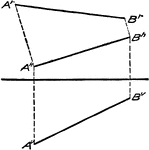### Construction of Geometric Revolution

"The distance of A and B below H is indicated on the V projection. Thus if to A∧h B∧h the perpendiculars…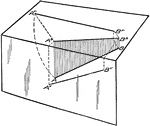### Revolutions into the Plane of Geometric Shapes

"The H projection of the line AB in the space is a line connecting the feet of all the perpendiculars…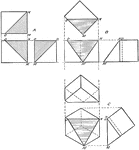### Successive Revolutions of Geometric Shapes

"A block revolved from its first position about an axis perpendicular to H through 45 degrees, then…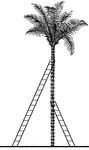### 2 Ladders Leaning Against a Tree

Illustration of 2 ladders leaning against opposite sides of a palm tree to form similar right triangles.…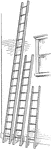### 3 Ladders Leaning Against a Wall

Illustration of 3 ladders leaning against the side of a building (wall) to form right triangles. The…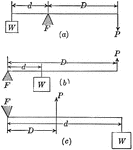### Lever and Fulcrum

Illustration of a lever with fulcrum F. W represents the weight lifted, P is the force that does the…### Hammer Used as a Lever

Illustration of a hammer being used as a lever. F represents the fulcrum.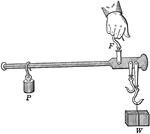### Steel-yard Used as a Lever

Illustration of an ordinary steel-yard being used as a lever. F represents the fulcrum. Weight P is…### Parallel Lines Cut by Transversals

Illustration that shows if two parallel lines are cut by three or more transversals that pass through…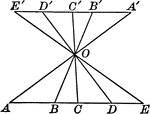### Parallel Lines Cut by Transversals

Illustration that shows if two parallel lines are cut by three or more transversals that pass through…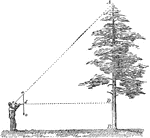### Measuring Tree Height

A man measuring the height of a tree by determining the angle and how far away he is standing.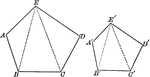### Similar Pentagons

Illustration of 2 similar pentagons.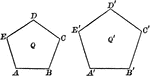### Similar Pentagons

Illustration of 2 similar pentagons.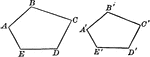### Similar Polygons (Pentagons) That Can Be Used To Show Proportionality

Illustration that shows similar polygons (pentagons) that can be used to show proportionality.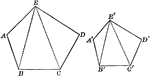### Similar Polygons (Pentagons) That Can Be Used To Show Proportionality

Illustration that shows similar polygons (pentagons) that can be used to show proportionality.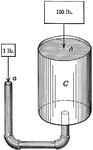### Hydraulic Machine Exerting Pressure

Illustration of a hydraulic machine. "A principle known as Pascal's Law states that pressure exerted…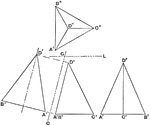### Triangle Pyramid Side Projections

The illustration showing the projection of the triangles from the triangle pyramid as shown on top.…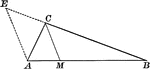### Angle Bisector of Triangle Dividing Opposite Side Into Proportionate Segments

Illustration to show that an angle bisector of a triangle divides the opposite side into segments which…### Exterior Angle Bisector of Triangle Dividing Opposite Side Into Proportionate Segments

Illustration to show that a bisector of a an exterior angle of a triangle divides the opposite side…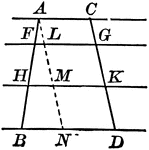### Parallel Lines Cut by Parallels, Corresponding Intercepts Proportional

Illustration to show that if parallel lines are cut by any number of parallels, the corresponding intercepts…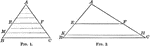### Triangle Divided by Parallel Lines to Form Proportionate Triangles

Illustration to show that if a line is drawn through two sides of a triangle parallel to the third side,…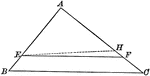### Triangle Divided by Parallel Lines to Form Proportionate Triangles

Illustration to show that if a line divides two sides of a triangle proportionally, it is parallel to…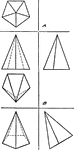### Revolution About Axis Forward or Back

"The pyramid be revolved about an axis perpendicular to V, the front view will be unchanged and may…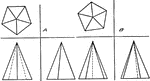### Revolution About Axis Perpendicular to H

The object can revolve around an axis (H) to create a projection on the other plane without changing…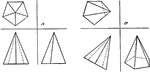### Revolution About Axis Perpendicular to V

"The pyramid be revolved about an axis perpendicular to V, the front view will be unchanged and may…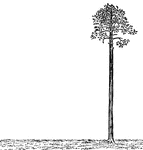### Palm Tree Perpendicular to Ground

Illustration of a palm tree that is perpendicular to the ground. The tree is perfectly straight, as…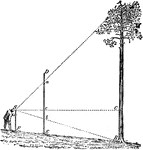### Using Proportions To Find Heights of Trees

Triangle diagram for measuring heights of trees using proportions.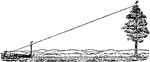### Using Proportions To Find Heights of Trees

Triangle diagram for measuring heights of trees using proportions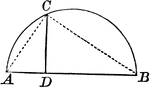### Right Triangle Inscribed in Semicircle Shows Mean Proportional

Right triangle inscribed in semicircle. Illustration shows that the perpendicular from any point in…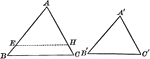### Mutually Equiangular Similar Triangles

Illustration that shows two mutually equiangular triangles are similar.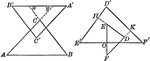### Similar Triangles With Respective Sides Parallel or Perpendicular

Illustration that shows that two triangles which have their sides respectively parallel, or respectively…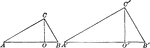### Similar triangles

Illustration of 2 similar triangles with altitudes drawn.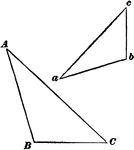### Similar Triangles

Illustration of two similar triangles, abc and ABC.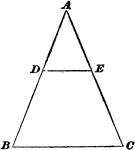### Similar Triangles

Illustration of a triangle divided by a parallel line to form two similar triangles.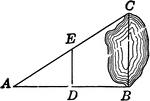### Similar Triangles for Distance Across Lake

Illustration of similar triangles used to find distance across a lake.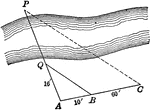### Similar Triangles for Distance Across Stream

Illustration of similar triangles used to find distance across a stream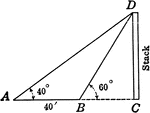### Similar Triangles for Height of Smokestack

Illustration of similar triangles used to find height of smokestack.### Similar Triangles With Altitudes Drawn

Illustration that shows two similar triangles with altitudes drawn.